# Title 40

## SECTION 53.54

### 53.54 Test for proper sampler operation following power interruptions.

§ 53.54 Test for proper sampler operation following power interruptions.

(a) Overview. (1) This test procedure is designed to test certain performance parameters of the candidate sampler during a test period in which power interruptions of various duration occur. The performance parameters tested are:

(i) Proper flow rate performance of the sampler.

(ii) Accuracy of the sampler's average flow rate, CV, and sample volume measurements.

(iii) Accuracy of the sampler's reported elapsed sampling time.

(iv) Accuracy of the reported time and duration of power interruptions.

(2) This test shall be conducted during operation of the test sampler over a continuous 6-hour test period during which the sampler's flow rate shall be measured and recorded at intervals not to exceed 5 minutes. The performance parameters tested under this procedure, the corresponding minimum performance specifications, and the applicable test conditions are summarized in table E-1 of this subpart. Each performance parameter tested, as described or determined in the test procedure, must meet or exceed the associated performance specification to successfully pass this test.

(b) Required test equipment. (1) Flow rate meter, suitable for measuring and recording the actual volumetric sample flow rate at the sampler downtube, with a minimum range of 10 to 25 L/min, 2 percent certified, NIST-traceable accuracy. Optional capability for continuous (analog) recording capability or digital recording at intervals not to exceed 5 minutes is recommended. While a flow meter which provides a direct indication of volumetric flow rate is preferred for this test, an alternative certified flow measurement device may be used as long as appropriate volumetric flow rate corrections are made based on measurements of actual ambient temperature and pressure conditions.

(2) Ambient air temperature sensor (if needed for volumetric corrections to flow rate measurements), with a resolution of 0.1 °C, certified accurate to within 0.5 °C, and continuous (analog) recording capability or digital recording at intervals not to exceed 5 minutes.

(3) Barometer (if needed for volumetric corrections to flow rate measurements), range 600 mm Hg to 800 mm Hg, certified accurate to 2 mm Hg, with continuous (analog) recording capability or digital recording at intervals not to exceed 5 minutes.

(4) Flow measurement adaptor (40 CFR part 50, appendix L, figure L-30) or equivalent adaptor to facilitate measurement of sample flow rate at the sampler downtube.

(5) Means for creating an additional pressure drop of 55 mm Hg in the sampler to simulate a heavily loaded filter, such as an orifice or flow restrictive plate installed in the filter holder or a valve or other flow restrictor temporarily installed in the flow path near the filter.

(6) Teflon sample filter, as specified in section 6 of 40 CFR part 50, appendix L (if required).

(7) Time measurement system, accurate to within 10 seconds per day.

(c) Calibration of test measurement instruments. Submit documentation showing evidence of appropriately recent calibration, certification of calibration accuracy, and NIST-traceability (if required) of all measurement instruments used in the tests. The accuracy of flow rate meters shall be verified at the highest and lowest pressures and temperatures used in the tests and shall be checked at zero and at least one flow rate within ±3 percent of 16.7 L/min within 7 days prior to use for this test. Where an instrument's measurements are to be recorded with an analog recording device, the accuracy of the entire instrument-recorder system shall be calibrated or verified.

(d) Test setup. (1) Setup of the sampler shall be performed as required in this paragraph (d) and otherwise as described in the sampler's operation or instruction manual referred to in § 53.4(b)(3). The sampler shall be installed upright and set up in its normal configuration for collecting PM samples. A sample filter and (or) the device for creating an additional 55 mm Hg pressure drop shall be installed for the duration of these tests. The sampler's ambient temperature, ambient pressure, and flow measurement systems shall all be calibrated per the sampler's operating manual within 7 days prior to this test.

(2) The inlet of the candidate sampler shall be removed and the flow measurement adaptor installed on the sample downtube. A leak check as described in the sampler's operation or instruction manual shall be conducted and must be properly passed before other tests are carried out.

(3) The inlet of the flow measurement adaptor shall be connected to the outlet of the flow rate meter.

(e) Procedure. (1) Set up the sampler as specified in paragraph (d) of this section and otherwise prepare the sampler for normal sample collection operation as directed in the sampler's operation or instruction manual. Set the sampler to automatically start a 6-hour sample collection period at a convenient time.

(2) During the entire 6-hour operational flow rate portion of the test, measure and record the sample flow rate with the flow rate meter at intervals not to exceed 5 minutes. If ambient temperature and pressure corrections are necessary to calculate volumetric flow rate, ambient temperature and pressure shall be measured at the same frequency as that of the certified flow rate measurements. Note and record the actual start and stop times for the 6-hour flow rate test period.

(3) During the 6-hour test period, interrupt the AC line electrical power to the sampler 5 times, with durations of 20 seconds, 40 seconds, 2 minutes, 7 minutes, and 20 minutes (respectively), with not less than 10 minutes of normal electrical power supplied between each power interruption. Record the hour and minute and duration of each power interruption.

(4) At the end of the test, terminate the sample period (if not automatically terminated by the sampler) and download all archived instrument data from the test sampler.

(f) Test results. At the completion of the sampling period, validate the test conditions and determine the test results as follows:

(1) Mean sample flow rate. (i) From the certified measurements (Qref) of the test sampler flow rate, tabulate each flow rate measurement in units of L/min. If ambient temperature and pressure corrections are necessary to calculate volumetric flow rate, each measured flow rate shall be corrected using its corresponding temperature and pressure measurement values. Calculate the mean flow rate for the sample period (Qref,ave) as follows:

Equation 6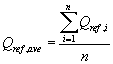where: n equals the number of discrete certified flow rate measurements over the 6-hour test period, excluding flow rate values obtained during periods of power interruption.

(ii)(A) Calculate the percent difference between this mean flow rate value and the design value of 16.67 L/min, as follows:

Equation 7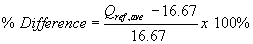(B) To successfully pass this test, the percent difference calculated in Equation 7 of this paragraph (f)(1)(ii) must be within ±5 percent.

(2) Sample flow rate regulation. (i) From the certified measurements of the test sampler flow rate, calculate the sample coefficient of variation of the discrete measurements as follows:

Equation 8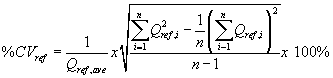(ii) To successfully pass this test, the calculated coefficient of variation for the certified flow rates must not exceed 2 percent.

(3) Flow rate measurement accuracy. (i) Using the mean volumetric flow rate reported by the candidate test sampler at the completion of the 6-hour test (Qind,ave), determine the accuracy of the reported mean flow rate as:

Equation 9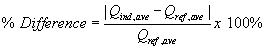(ii) To successfully pass this test, the percent difference calculated in Equation 9 of this paragraph (f)(3) shall not exceed 2 percent.

(4) Flow rate CV measurement accuracy. (i) Using the flow rate coefficient of variation indicated by the candidate test sampler at the completion of the 6-hour test (%CVind), determine the accuracy of the reported coefficient of variation as:

Equation 10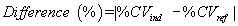(ii) To successfully pass this test, the absolute difference in values calculated in Equation 10 of this paragraph (f)(4) must not exceed 0.3 (CV%).

(5) Verify that the sampler properly provided a record and visual display of the correct year, month, day-of-month, hour, and minute with an accuracy of ±2 minutes, of the start of each power interruption of duration greater than 60 seconds.

(6) Calculate the actual elapsed sample time, excluding the periods of electrical power interruption. Verify that the elapsed sample time reported by the sampler is accurate to within ±20 seconds for the 6-hour test run.

(7) Calculate the sample volume as Qref.ave multiplied by the sample time, excluding periods of power interruption. Verify that the sample volume reported by the sampler is within 2 percent of the calculated sample volume to successfully pass this test.

(8) Inspect the downloaded instrument data from the test sampler and verify that all data are consistent with normal operation of the sampler.

[62 FR 38799, July 18, 1997; 63 FR 7714, Feb. 17, 1998, as amended at 71 FR 61291, Oct. 17, 2006]